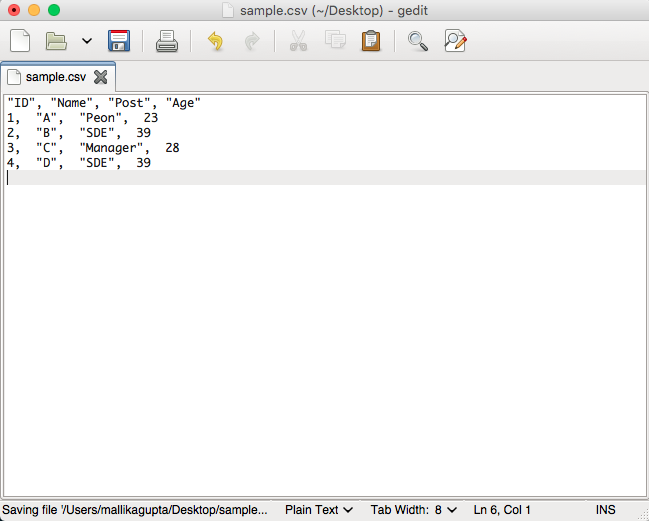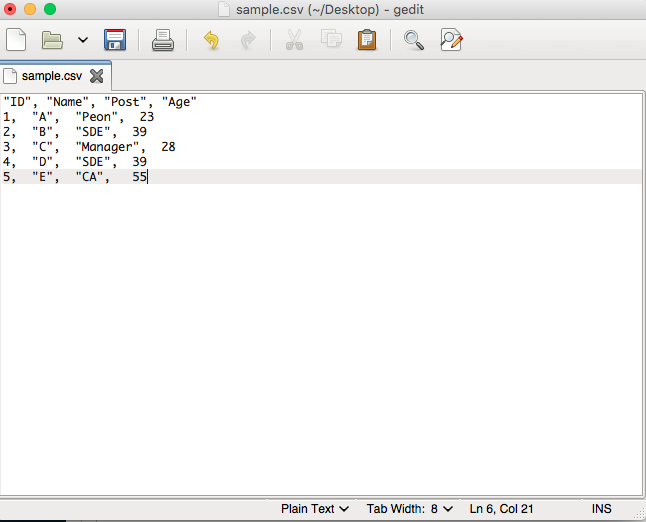# Append row to CSV using R

• Last Updated : 28 Apr, 2021

In this article, we will see how to append rows to a CSV file using R Programming Language.

By default, the write.csv() function overwrites entire file content. In order to append the data to a CSV File, use the write.table() method instead and set the parameter, append = TRUE. The write.table method prints its required argument x, upon conversion of the .csv file into the data frame to a file or connection.

Syntax:

write.table(x, file = “”, append = FALSE, quote = TRUE, sep = ” “, row.names = TRUE, col.names = TRUE)

Parameters:

• x : The row data in the data frame object
• file : The file to append row to
• sep : The field separator string, that is within each row of x values are separated by this separator.

In case the file is empty, the contents are written on to the .csv file upon creation within the same directory. The following code illustrates the applicability of write.table() method on an empty .csv file.

Example

## R

 `# defining the data for the csv file ``# data is organised into 4 columns``data = ``data.frame``(ID = 1:4, Name = ``c``(``"A"``,``"B"``,``"C"``,``"D"``),``                  ``Post=``c``(``"Peon"``,``"SDE"``,``"Manager"``,``"SDE"``), ``                  ``Age = ``c``(23,39,28,39))`` ` `# write data to a sample.csv file``write.table``(data, file = ``"sample.csv"``) `

OutputThe contents are written onto the empty sample.csv file

The following snippet shows how to append a row to the CSV file already composed of a few rows. The changes are made to the specified CSV file, and upon each operation, one-row count is incremented.

Example:

## R

 `# defining a row ``row <- ``data.frame``(``'1'``, ``'A'``, ``'Manager'``, ``'24'``)`` ` `# sample csv name``csv_fname = ``"sample.csv"`` ` `# writing row in the csv file``write.table``(row, file = csv_fname, sep = ``","``,``            ``append = ``TRUE``, quote = ``FALSE``,``            ``col.names = ``FALSE``, row.names = ``FALSE``)`

Output“sample.csv” : After the execution of Python script

My Personal Notes arrow_drop_up